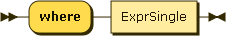### Where clauses

JSONiq follows the W3C standard for where clauses. The following explanations, provided as an informal summary for convenience, are non-normative.

Figure 55. WhereClauseWhere clauses are used for filtering (selection operator in the relational algebra).

For each incoming tuple, the expression in the where clause is evaluated to a boolean (possibly converting an atomic to a boolean). if this boolean is true, the tuple is forwarded to the next clause, otherwise it is dropped.

The following query corresponds to "SELECT series FROM captains WHERE name = 'Kathryn Janeway'".

Example 122. A where clause.

```for \$x in collection("captains")
where \$x.name eq "Kathryn Janeway"
return \$x.series
```

Result (run with Zorba): [ "The next generation", "Voyager" ]